## Introduction

• Several different deep learning frameworks exist today, each with their own strengths, weaknesses, and user base. In this topic, we’ll focus on the two most popular frameworks today: TensorFlow and PyTorch.
• The main difference between those frameworks is - code notwithstanding - the way in which they create and run computations. In general, deep learning frameworks represent neural networks as computational graphs. A sample TensorFlow Computational Graph is shown below: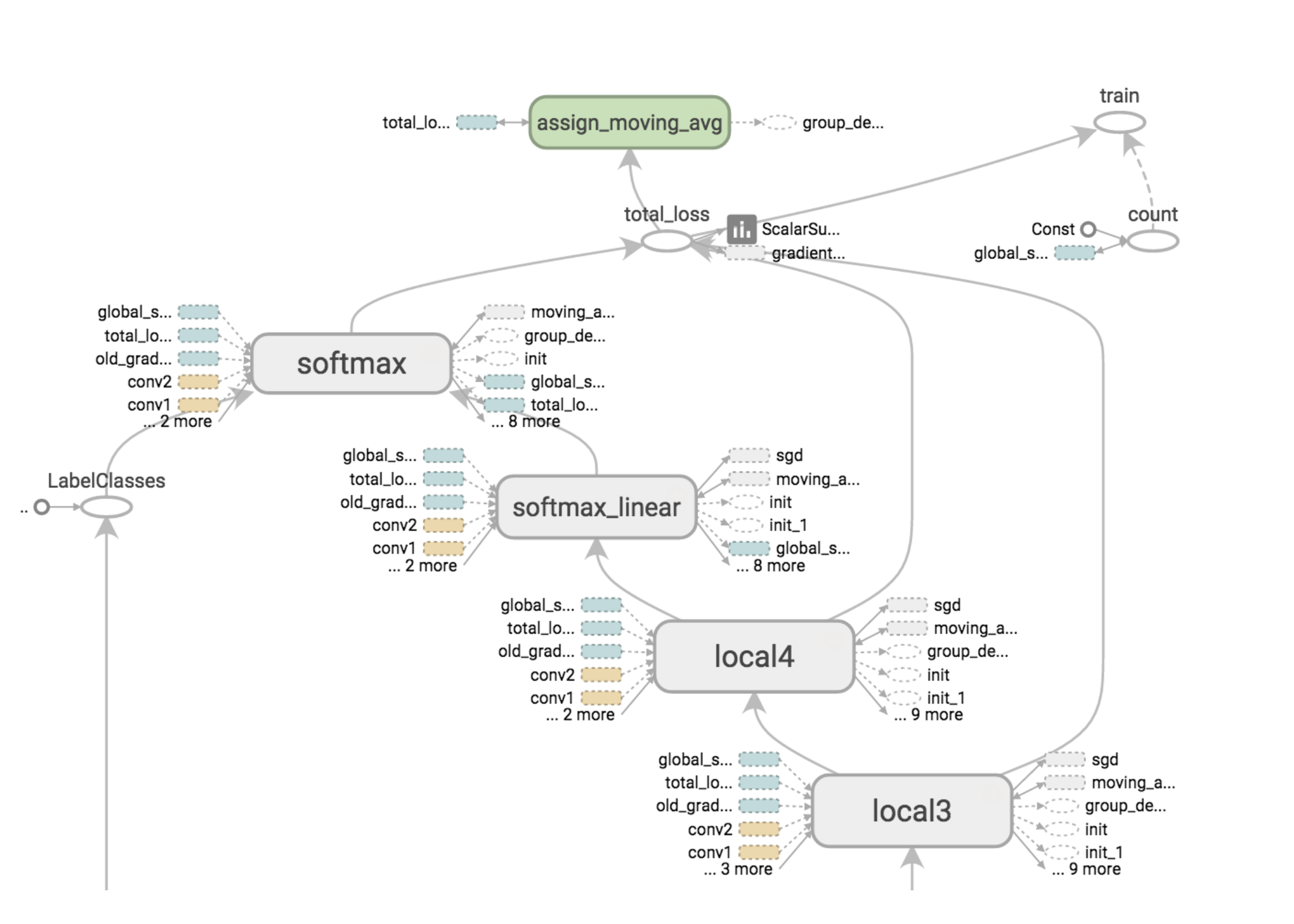• Our variables, such as the weights, biases, and loss function, are graph nodes defined before training. During training, the graph is run to execute the computations.
• TensorFlow and PyTorch, our two chosen frameworks, handle this computational graph differently. In TensorFlow, the graph is static. That means that we create and connect all the variables at the beginning, and initialize them into a static (unchanging) session. This session and graph persists and is reused: it is not rebuilt after each iteration of training, making it efficient. However, with a static graph, variable sizes have to be defined at the beginning, which can be non-convenient for some applications, such as NLP with variable length inputs.
• On the contrary, PyTorch uses a dynamic graph. That means that the computational graph is built up dynamically, immediately after we declare variables. This graph is thus rebuilt after each iteration of training. Dynamic graphs are flexible and allow us modify and inspect the internals of the graph at any time. The main drawback is that it can take time to rebuild the graph. Either PyTorch or TensorFlow can be more efficient depending on the specific application and implementation.
• Recently, TensorFlow 2.0 was released. This new TensorFlow uses dynamic graphs as well. We’ll take a glance at it in the section on .
• Now, let’s cover the main topics:

1. We’ll walk through how to build a full TensorFlow deep learning pipeline from scratch. A PyTorch notebook is also available as a comparison.
2. We will compare and contrast PyTorch vs. TensorFlow vs. TensorFlow 2.0 code.

## Deep Learning Pipeline

• Here is a list of steps to implement a deep learning pipeline:

2. Load and pre-process the dataset.
3. Define the model.
4. Define the loss function and optimizer.
5. Define the evaluation metric.
6. Train the network on the training data.
7. Report results on the train and test data.
• Let’s look at a TensorFlow notebook vs. a PyTorch notebook in Colab to see how to implement the above steps:

## PyTorch or TensorFlow?

• Both frameworks have their pros and cons. We also mention TensorFlow v1 in our discussions (even though TensorFlow v2 has now been around for a while) because a lot of legacy code with v1 still exists.
• PyTorch has long been the preferred deep-learning library for researchers, while TensorFlow is much more widely used in production.

### PyTorch

#### (+)

• Younger, but also well documented and fast-growing community.
• More pythonic and NumPy-like approach, designed for faster prototyping and research.
• Automatic differentiation using Autograd to compute the backward pass given a forward pass of a network (note that TensorFlow v2 has this capability).
• Uses eager execution mode by default (i.e., dynamic graph), compared to TensorFlow v1’s static graph paradigm.
• Follows the channel-first (also called spatial-first) convention, i.e., $$N, C, H, W$$ for images which makes it faster than TensorFlow’s channel-last convention.
• Easy to debug and customize.

#### (-)

• Need to manually zero out gradients using zero_grad() at the start of a new mini-batch.
• this is because loss.backward() accumulates gradients (and doesn’t overwrite them), and you don’t want to mix up gradients between mini-batches.
• Using a GPU requires code changes to copy your model’s parameters/tensors over to your GPU.

### TensorFlow

#### (+)

• Mature, most of the models and layers are already implemented in the library (has Keras builtin at tf.keras).
• Built for large-scale deployment and is the tool-of-choice in the industry.
• Has some very useful tools like TensorBoard for visualization (although TensorBoardX now exists for PyTorch).
• TensorFlow v2 uses eager execution/dynamic graphs (but TensorFlow v1) just like PyTorch v1.
• No need to manually zero out gradients for the backward pass.
• Transparent use of the GPU.

#### (-)

• Some ramp-up time is needed to understand some of the concepts (session, graph, variable scope, etc.), especially with TensorFlow v1.
• Follows the channel-last convention, i.e., $$N, H, W, C$$ for images due to legacy reasons, which makes it slower.
• Can be harder to debug.

## Comparing PyTorch vs TensorFlow with code

• The main differences between these frameworks are in the way in which variables are assigned and the computational graph is run. TensorFlow 2.0 works similarly to PyTorch.

• TensorFlow v1 and v2: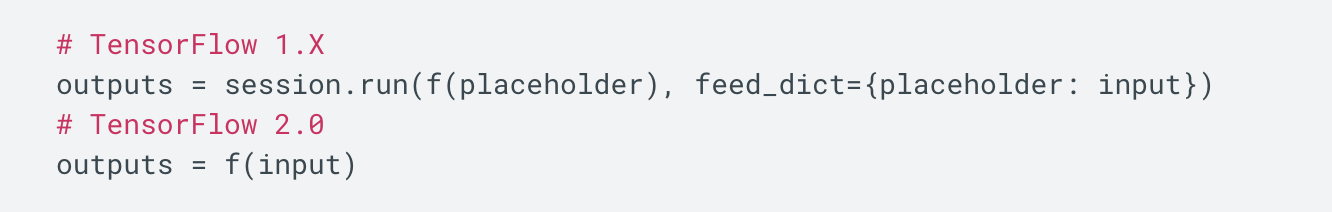• With TensorFlow v2.0, we don’t initialize and run a session with placeholders. Instead, the computational graph is built up dynamically as we declare variables, and calling a function with an input runs the graph and provides the output, like a standard Python function.

• TensorFlow v1: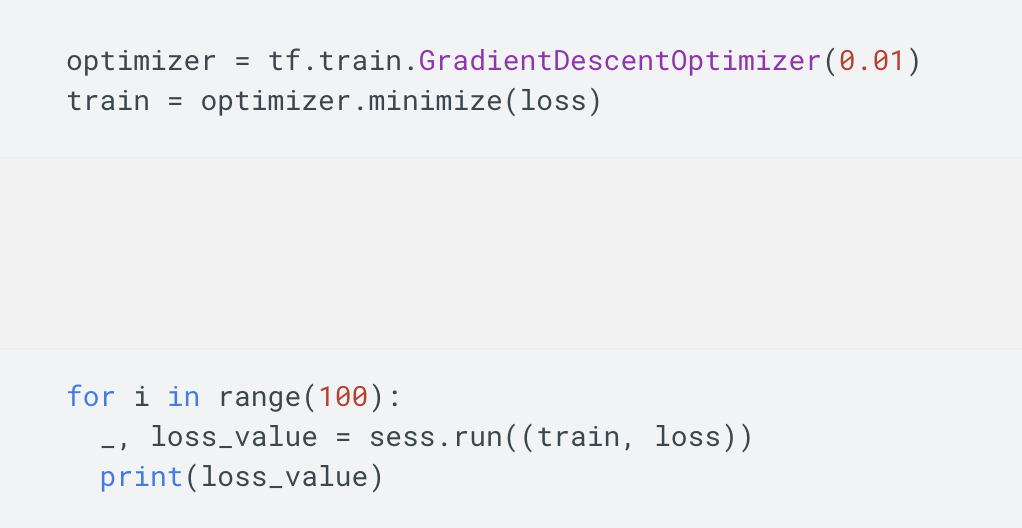• TensorFlow v2: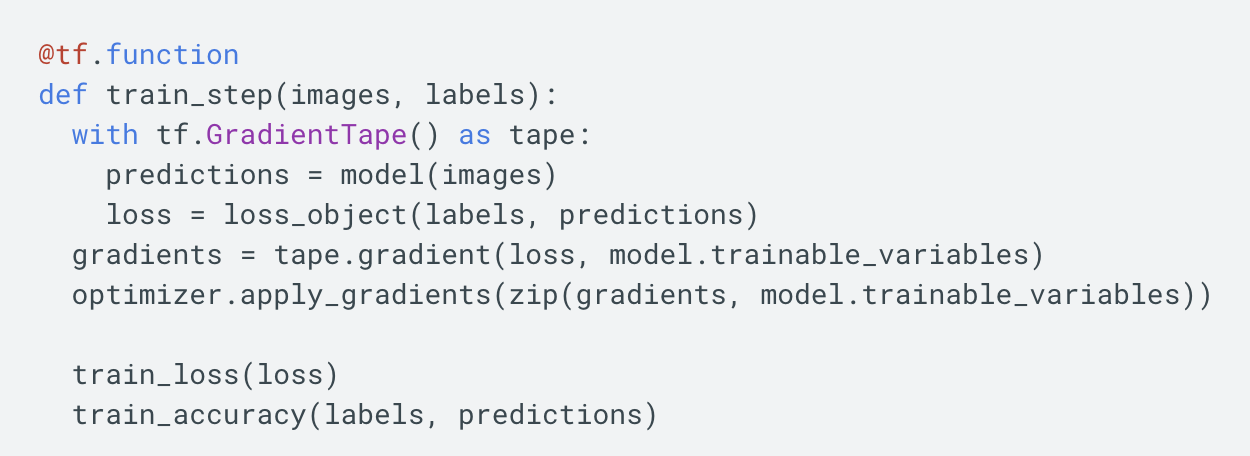• PyTorch: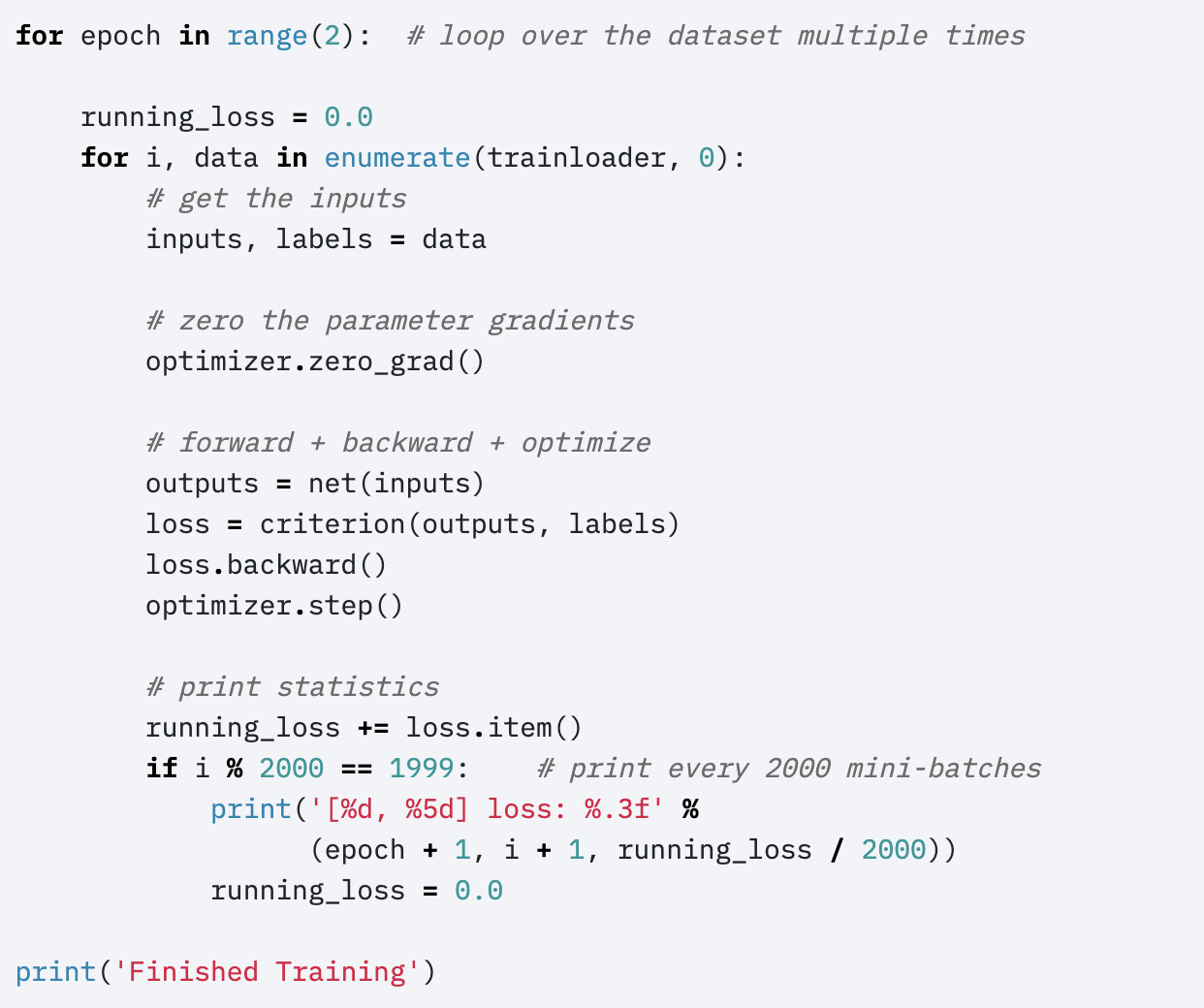• So which one will you choose? :)

## Citation

If you found our work useful, please cite it as:

@article{Chadha2020DistilledPyTorchvsTensorFlow,
title   = {PyTorch vs. TensorFlow},